# Underscore.js | _.chunk()

_.chunk() function:

• It is used to split a passed array into a number of arrays containing the number of elements given in the second parameter of the _.chunk() function.
• We can convert a single array into a number of arrays using this function.
• Also, the number of elements which the resultant array will contain should be given in the second parameter.

Syntax:

`_.chunk(array, length) `

Parameters:
It takes two arguments:

• The array
• The length of the resultant arrays

Return value:
It returns the number of arrays that are formed after the split.

Examples:

1. Passing a list of numbers to _.chunk() function:
The ._chunk() function takes the element from the list one by one and forms an array until the number of elements in the reusltant array is equal to the given number in the second parameter. Then, if the elements in the given array are still present then it forms another array in the result. Finally the total number of arrays formed is equal to the number of elements in the passed array divided by the number given in the second element.

 `` `<``html``> ` `  `  `<``head``> ` `    ``<``script` `src` `=  ` `"https://cdnjs.cloudflare.com/ajax/libs/underscore.js/1.9.1/underscore-min.js"` `> ` `    `` ` ` ` `  `  `<``body``> ` `    ``<``script` `type``=``"text/javascript"``> ` `        ``console.log(_.chunk([1, 2, 3, 4, 5, 6], 2)); ` `    `` ` ` ` `  `  ` `

Output: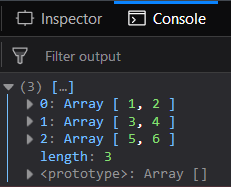2. Passing a larger array size to the _.chunk() function:
If we pass a larger sized number in the second parameter then also it works in the same way. Like here the number of elements is given is 3. So, we form the number of arrays. Each array have three elements in the same order it is present in the passed array.

 `` `<``html``> ` `  `  `<``head``> ` `    ``<``script` `src` `=  ` `"https://cdnjs.cloudflare.com/ajax/libs/underscore.js/1.9.1/underscore-min.js"` `> ` `    `` ` ` ` `  `  `<``body``> ` `    ``<``script` `type``=``"text/javascript"``> ` `        ``console.log(_.chunk([1, 2, 3, 4, 5, 6, 7, 8, 9], 3)); ` `    `` ` ` ` `  `  ` `

Output: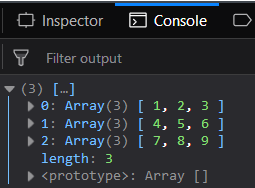3. Passing a list of characters to the _.chunk() function:
Even if we pass the array containing alphabets .i.e., string of characters rather than numbers then also it will work in the same manner. This implies that the _.chunk() function does not distinguish between numbers, characters etc. Also, since here the number passed in the second parameter is a two but the passed array has five elements. Therefore, the last element will have only one element. It will not give any error.

 `` `<``html``> ` `  `  `<``head``> ` `    ``<``script` `src` `=  ` `"https://cdnjs.cloudflare.com/ajax/libs/underscore.js/1.9.1/underscore-min.js"` `> ` `    `` ` ` ` `  `  `<``body``> ` `    ``<``script` `type``=``"text/javascript"``> ` `        ``console.log(_.chunk(['HTML', 'CSS', 'JS', 'AJAX', 'PEARL'], 2)); ` `    `` ` ` ` `  `  ` `

Output: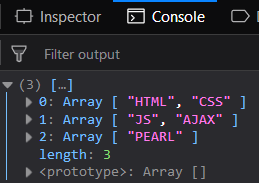4. Having the same number of array as the number of elements to the _.chunk() function:
We can even obtain the result the same umber as the elements passed in the array. This makes the use of _.chunk() function more convenient. For this we just need to pass the second parameter as 1. This implies that the size of each array will be one.

 `` `<``html``> ` `  `  `<``head``> ` `    ``<``script` `src` `=  ` `"https://cdnjs.cloudflare.com/ajax/libs/underscore.js/1.9.1/underscore-min.js"` `> ` `    `` ` ` ` `  `  `<``body``> ` `    ``<``script` `type``=``"text/javascript"``> ` `        ``console.log(_.chunk(['HTML', 'CSS', 'JS', 'AJAX', 'PEARL'], 1)); ` `    `` ` ` ` `  `  ` ` ` `

Output: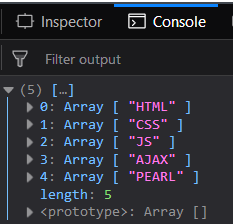NOTE:
These commands will not work in Google console or in firefox as for these additional files need to be added which they didn’t have added.

 `` `<``script` `type``=``"text/javascript"`  `src` `=``"https://cdnjs.cloudflare.com/ajax/libs/underscore.js/1.9.1/underscore-min.js"``> ` ` `

An example is shown below: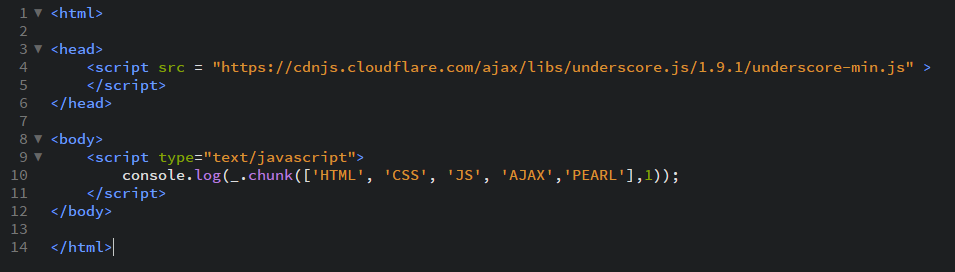My Personal Notes arrow_drop_upCheck out this Author's contributed articles.

If you like GeeksforGeeks and would like to contribute, you can also write an article using contribute.geeksforgeeks.org or mail your article to contribute@geeksforgeeks.org. See your article appearing on the GeeksforGeeks main page and help other Geeks.

Please Improve this article if you find anything incorrect by clicking on the "Improve Article" button below.

Improved By : nidhi_biet

Article Tags :

Be the First to upvote.

Please write to us at contribute@geeksforgeeks.org to report any issue with the above content.